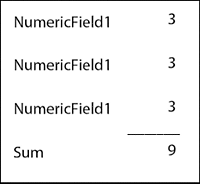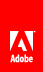# Calculating the field sums

This example demonstrates how to calculate the sums of fields located at different levels of the form hierarchy when the form filler opens the form in a client application, such as Acrobat Professional, Adobe Reader, or HTML client.

View full size graphicTo see this scripting example and others, visit tvisit the Developer Center .

#### Scripting for calculating the sum of repeating fields in a form

To calculate the sum of repeating fields in a form, you add a calculate event to the Sum field:

```    var fields = xfa.resolveNodes("NumericField1[*]");

var total = 0;
for (var i=0; i <= fields.length-1; i++) {
total = total + fields.item(i).rawValue;
}

this.rawValue = total;```

#### Scripting for calculating the sum of repeating fields

Similarly, to calculate the sum of repeating fields, you add a calculate event to the Sum field:

```    var fields = xfa.resolveNodes("detail[*].NumericField1");

var total = 0;
for (var i=0; i <= fields.length-1; i++) {
total = total + fields.item(i).rawValue;
}

this.rawValue = total;```

#### Scripting to calculate the sum of the fields on the page

To calculate the sum of the fields on the page, you add a calculate event to the Sum field:

```    var fields = xfa.layout.pageContent(0 , "field", 0);

var total = 0;
for (var i=0; i <= fields.length-1; i++) {
if (fields.item(i).name == "NumericField1") {
total = total + fields.item(i).rawValue;
}
}

this.rawValue = total;```// Ethnio survey code removed